# A) Show that Σ.1X, and Σηι x? are jointly sufficient statistics for two un known parameters of th...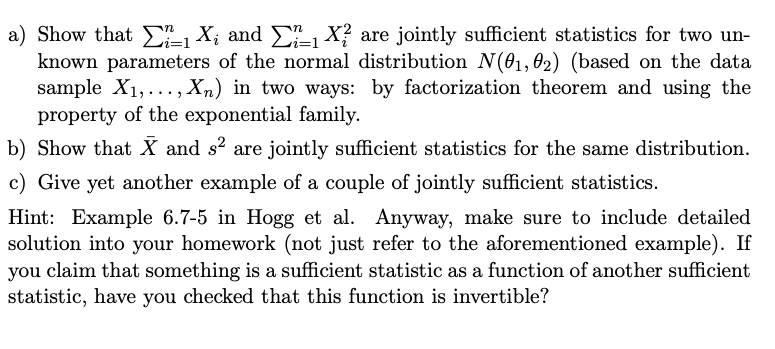a) Show that Σ.1X, and Σηι x? are jointly sufficient statistics for two un known parameters of the normal distribution N(01,02) (based on the data sample X1,..., Xn) in two ways: by factorization theorem and using the property of the exponential family. b) Show that X and s2 are jointly sufficient statistics for the same distribution. c) Give yet another example of a couple of jointly sufficient statistics. Hint: Example 6.7-5 in Hogg et al. Anyway, make sure to include detailed solution into your homework (not just refer to the aforementioned example). If you claim that something is a sufficient statistic as a function of another sufficient statistic, have you checked that this function is invertible?

First I define the factorization theorem and exponential familly. Then using these two I derive the sufficient statistics for each of the cases.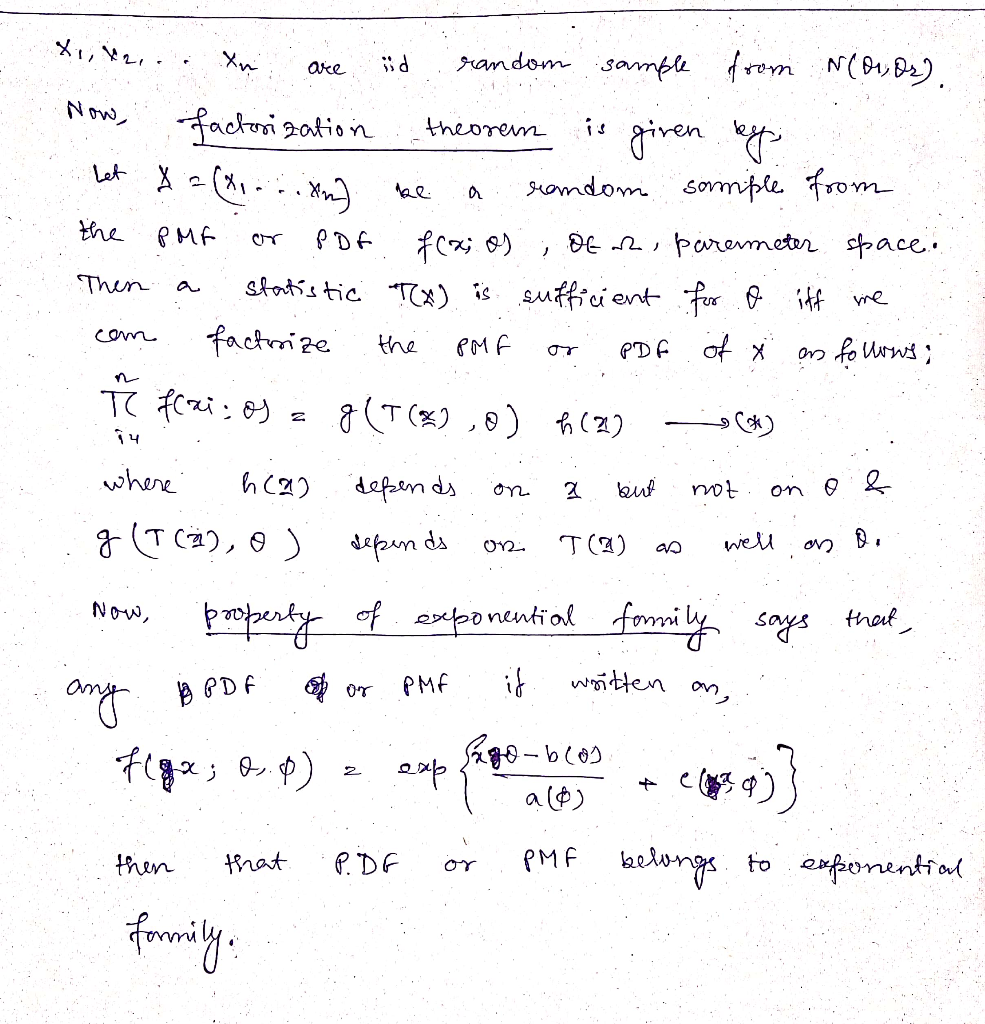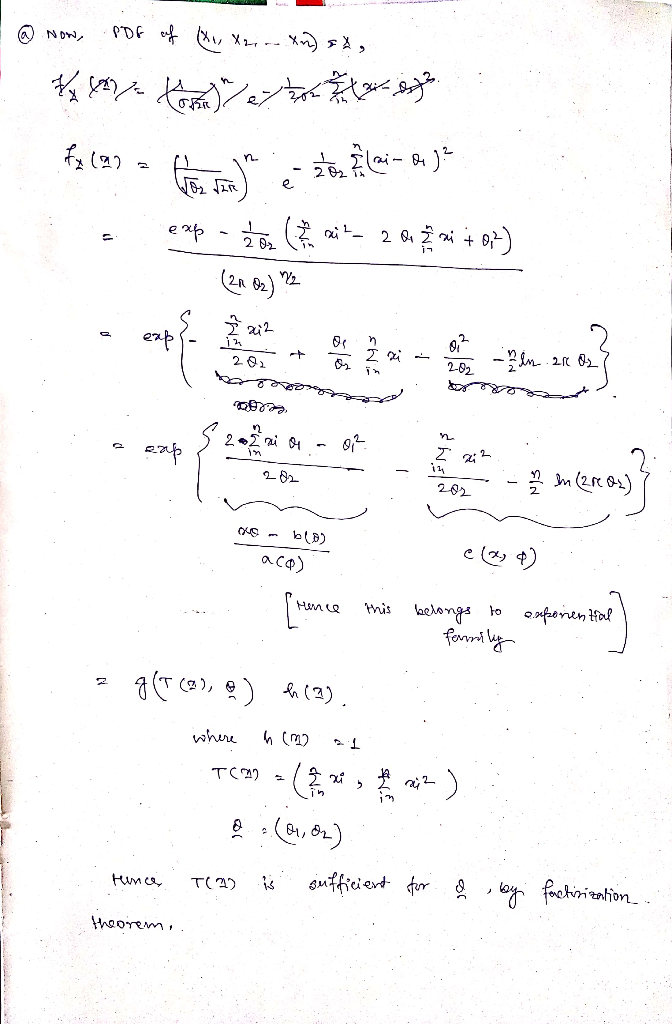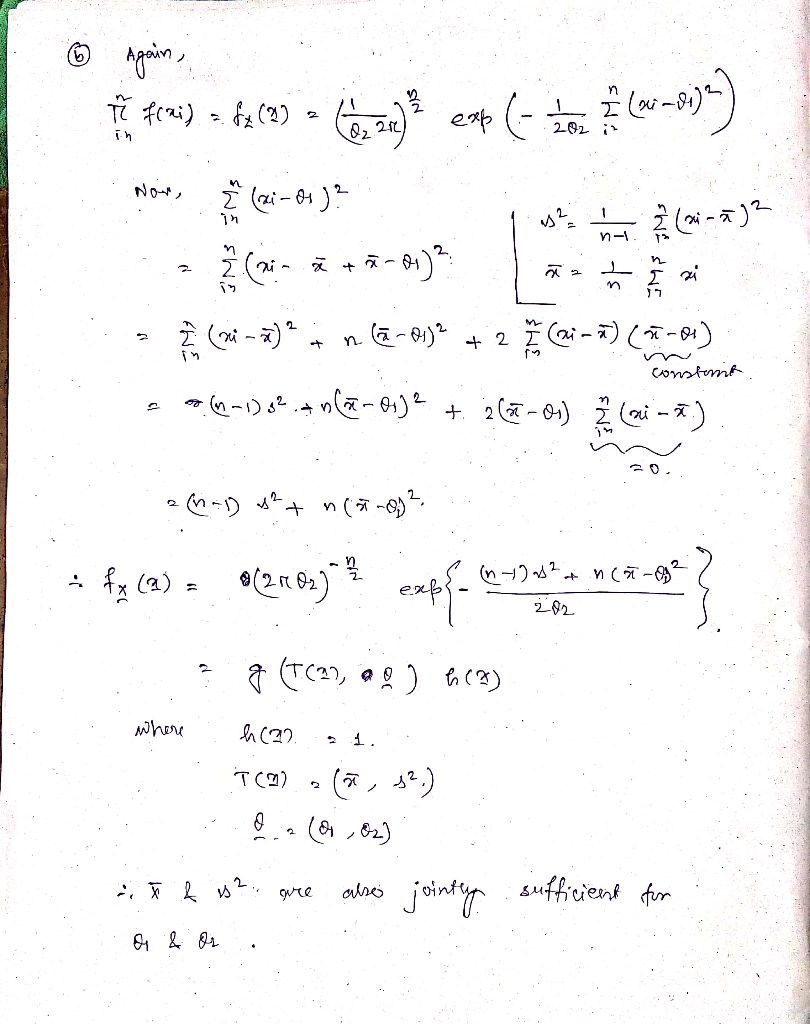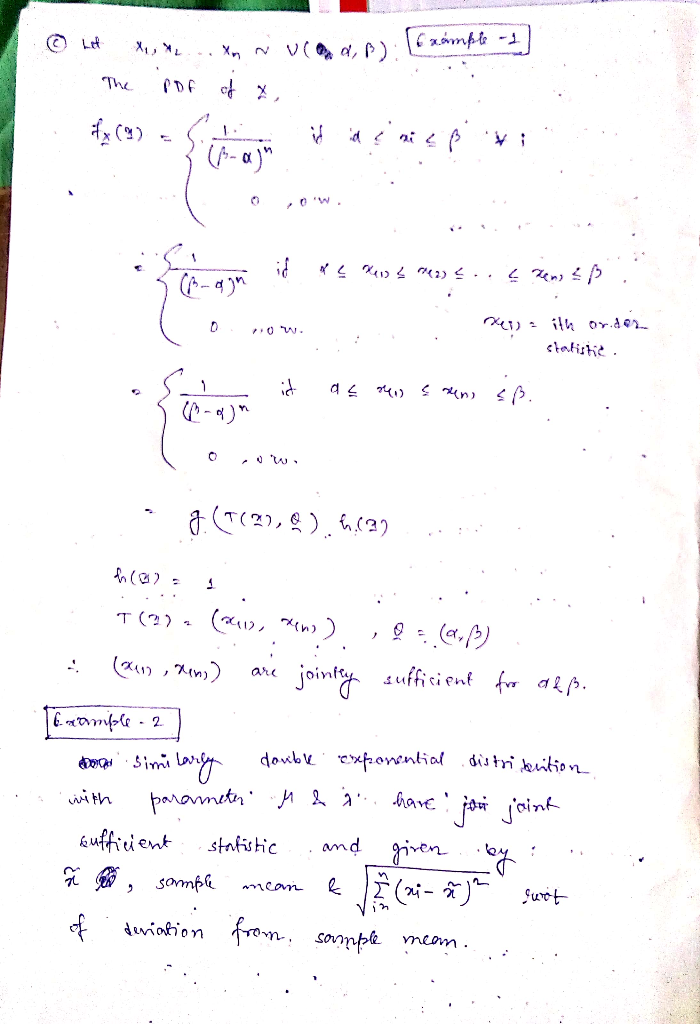##### Add Answer of: A) Show that Σ.1X, and Σηι x? are jointly sufficient statistics for two un known parameters of th...
More Homework Help Questions Additional questions in this topic.